Sometimes we wonder about the nature of sampling and its effects on group mean estimation. This code was put together to demonstrate the effects of small and large sample sizes on the estimation of means and the testing of significant differences between means.
This is probably not the cleanest way to run the demonstration, but I think it’s pretty cool anyway. A better way would be to plot a forced-normal distribution rather than a density function, but I’m not sure how to do that yet.
Okay, here we go…
First load some packages and clear the workspace

library(plyr)
library(ggplot2)
rm(list=ls())

Size of the each population sample

x <- 1:500

Establish a random seed so that this specific plot can be re-generated

set.seed(13)

Set up an explicit difference between two samples a and b;
the difference will be symmetrical around 0

mean.diff <- 0.0
sdev <- 4
a <- rnorm(n=length(x), mean = 0-(mean.diff/2), sd = sdev)
b <- rnorm(n=length(x), mean = 0+(mean.diff/2), sd = sdev)

# quick histogram
hist(a)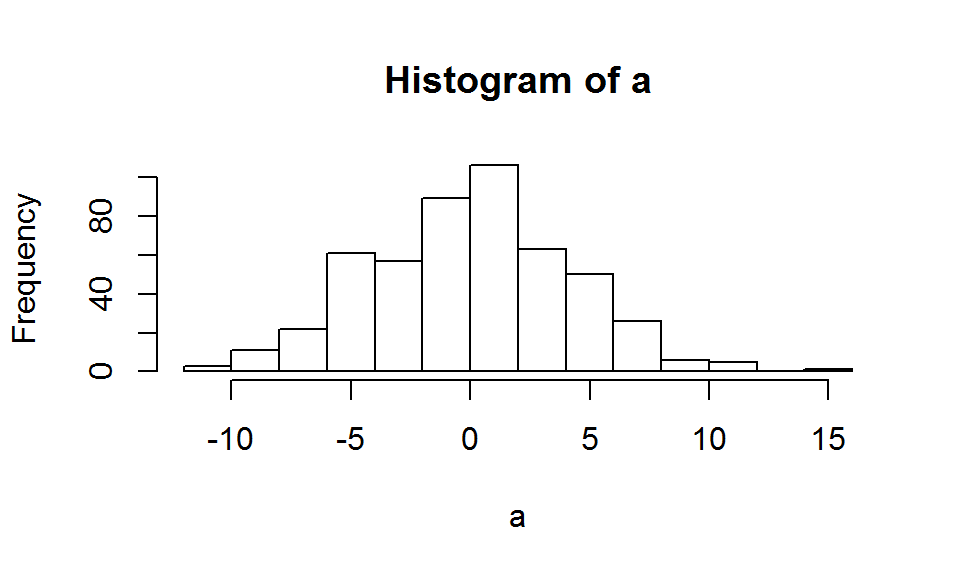hist(b)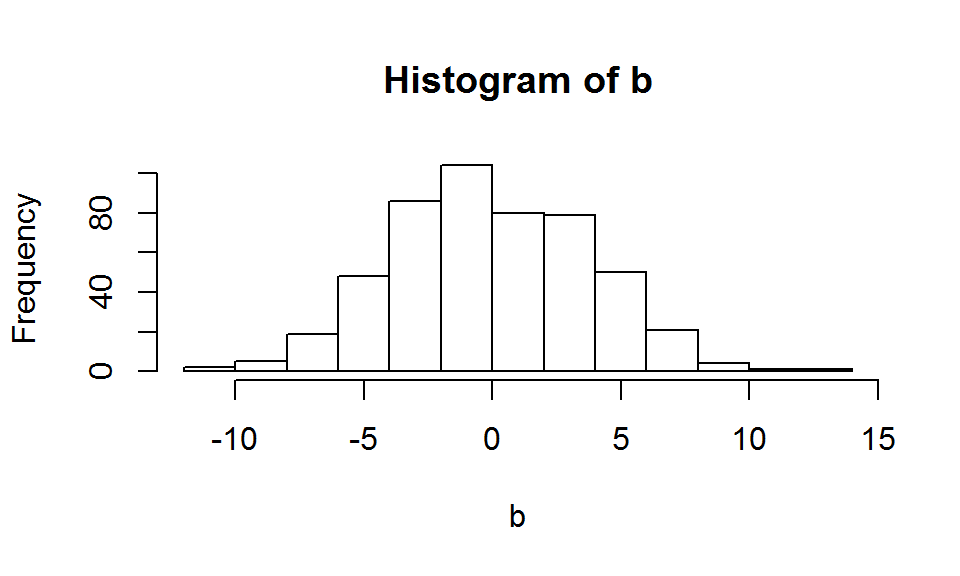Function: takes two arguments - a test name, and samples.
Samples determines the number of samples drawn from two previously created distributions (a and b) and does some basic analysis of those samples test.name is only a value added to the output to keep track of this iteration in the case of multiple tests with the same number of samples.

distrib.analysis <- function(test.name, samples){
a.sample <- sample(a, size = samples)
b.sample <- sample(b, size = samples)
mean.a <- mean(a.sample)
mean.b <- mean(b.sample)
sd.a <- sd(a.sample)
sd.b <- sd(b.sample)

obs <- c(a.sample, b.sample)
group <- c(rep("A",length(a.sample)), rep("B",length(b.sample)))
means <- c(rep(mean.a,length(a.sample)), rep(mean.b,length(b.sample)))
stdev <- c(rep(sd.a,length(a.sample)), rep(sd.b,length(b.sample)))
t.val <- t.test(a.sample, a.sample)$statistic p.val <- t.test(a.sample, b.sample)$p.value
temp <- data.frame(test.name, samples, group, obs, means, stdev, t.val, p.val)
return(temp)
}

Create a data frame that includes two lists:
an index of test number,
and a sample size corresponding to that test number.

### To “re-run” the sample, start here and run until the end of the script.

small.sample.size <- 10
large.sample.size <- 100
sample.sizes <- c(rep(small.sample.size, 15),rep(large.sample.size, 5))
test.number <- 1:length(sample.sizes)
sample.info <- data.frame(test.number, sample.sizes)

# explicitly code a variety of sample sizes using any of the various methods
sample.sizes2 <- c(5,7,10,15,20,25,30,35,50,75,100, 150)
# sample.sizes2 <- round(seq(5,max(x), length.out = 12),0)
test.number2 <- 1:length(sample.sizes2)
sample.info2 <- data.frame(test.number=test.number2, sample.sizes=sample.sizes2)

Goal: to supply the function with a vector of arguments to test.name and samples
apply the function distrib.analysis with the input arguments being the elements of sample.info[test.name], and sample.info[samples]

Generate the sample data from the selection of small and large sample sizes

all.data <- suppressWarnings(with(sample.info,
rbind.fill(Map(distrib.analysis,
test.name = test.number,
samples = sample.sizes))))

# do the same thing for the explicitly coded sample sizes
all.data2 <- suppressWarnings(with(sample.info2,
rbind.fill(Map(distrib.analysis,
test.name = test.number,
samples = sample.sizes))))

# code for standard p value threshold
all.data$signif <- ifelse(all.data$p.val < 0.05, "yes","no")
all.data2$signif <- ifelse(all.data2$p.val < 0.05, "yes","no")

Summary of group means, whether each comparison is significant, etc

all.data.info <- unique(all.data[!(names(all.data) %in% "obs")])
all.data.info2 <- unique(all.data2[!(names(all.data2) %in% "obs")])

Finally, plot the density distribution functions for the small-n groups and the large-n groups.

small.and.large <- ggplot(all.data)+
# big points to indicate significance threshold
geom_point(aes(shape=signif, fill=signif), x=sdev*1.8, y=0.1, size=6)+
# points for individual observations
geom_point(aes(x=obs, color=group, y=as.numeric(group)*0.03))+
scale_shape_manual(values = c(4,21))+
scale_fill_manual(values = c("white","black"))+
scale_color_brewer(palette = "Set1")+
geom_density(aes(x=obs, color=group), alpha=0.8)+
# vertical lines at the sample means
geom_vline(aes(xintercept=means, color=group), linetype="dashed")+
# vertical line at the pupilation mean
geom_vline(xintercept=0, color="black", linetype="solid")+
# indicate the number of samples in each facet
geom_text(aes(label=samples), x=-sdev*1.8, y=0.1)+
theme_bw()+
labs(x="Observations", y="Distribution Density")+
ggtitle(paste0("Mean difference: ", mean.diff, ", st.dev: ",sdev))+
facet_wrap(~test.name)
small.and.large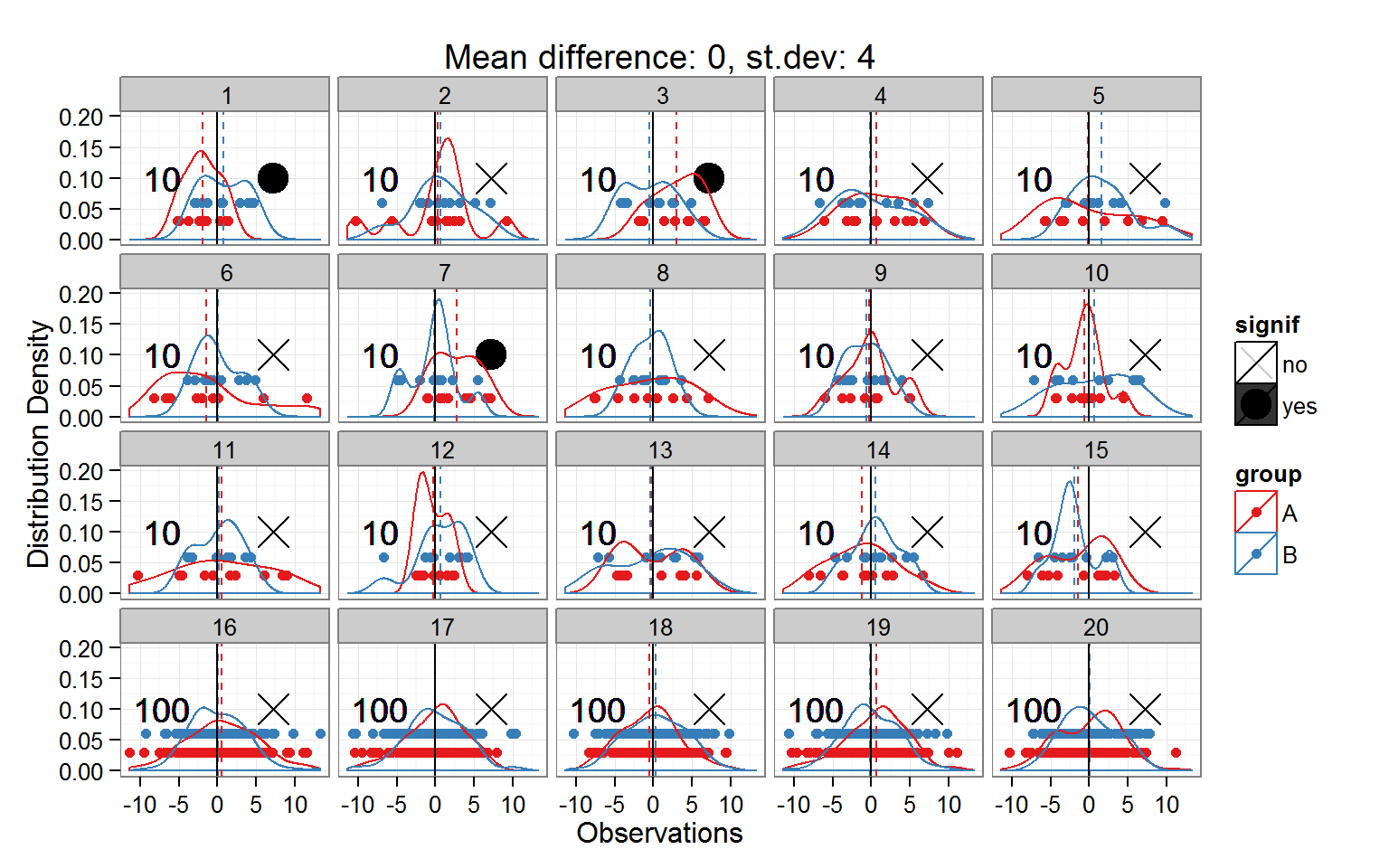See how some of the small sample size groups have spurious “significant” differences!
We know that it is spurious because we explicitly set the means of each group to be exactly equal, and with an equal amount of variance.
The randomness of the sample caused some observations to be chosen even though they were not representative of the group mean. That’s how it works.

Now let’s create a similar plot, but use a different data set - the one with explicitly declared sample sizes

explicit.sizes <- small.and.large %+% all.data2
explicit.sizes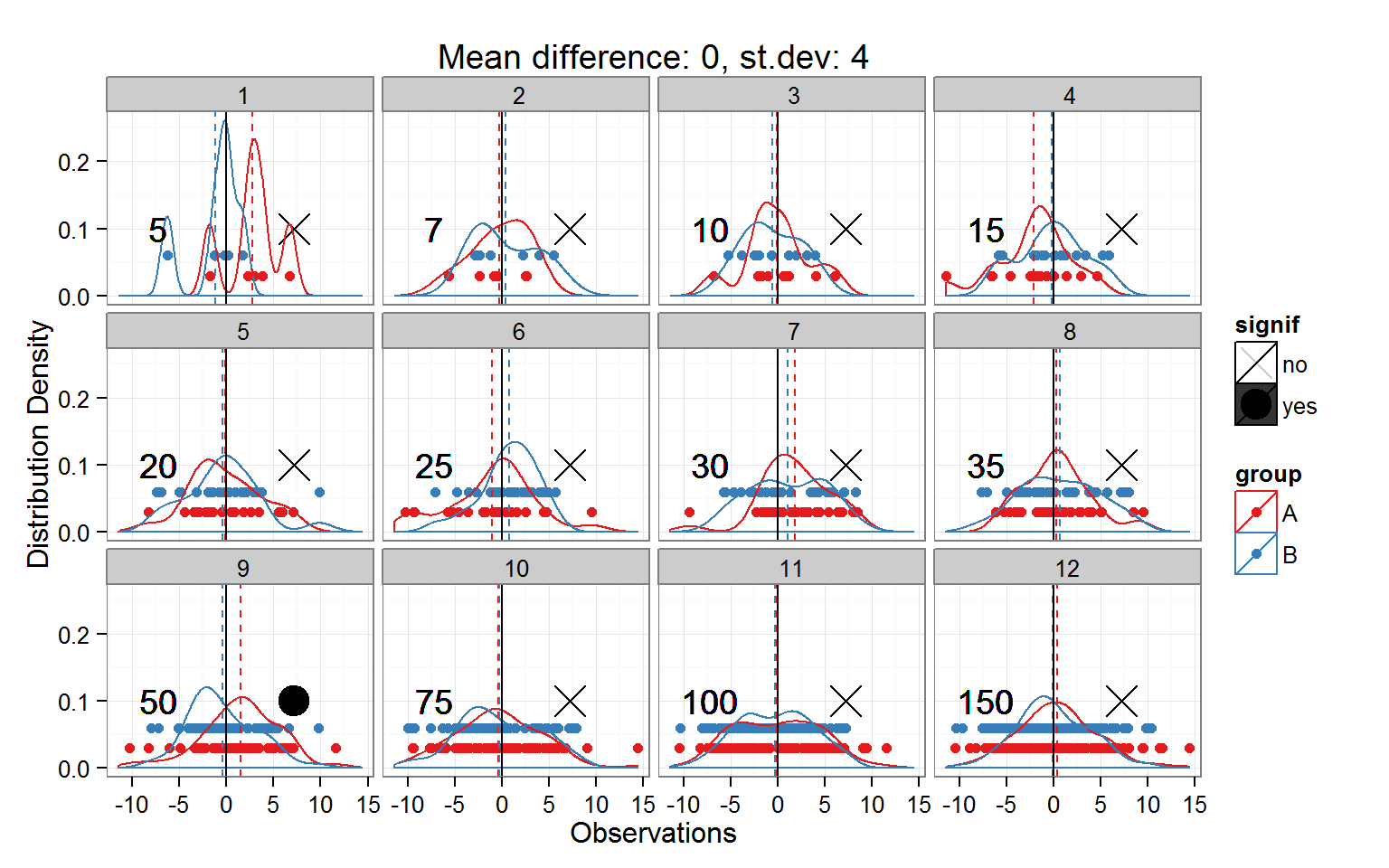Not as many spurious significances, but you can see that some of those small-n distributions do NOT look normal at all.
Frankly, they look mis-shapen and gooey. In the panels with a large number of observations, the distributions look more normal-shaped.

Remember, the common parametric tests of significance operate under the assumption that the distributions are normal. When we collect a small number of observations, we potentially fall short of acquiring enough evidence to support that assumption.

To verify that this method can actually detect differences when they are real, Now let’s run the same code as above, except I will set the mean difference to be something real (not zero). The goal is to see if the statistical analysis will detect the true difference that is being declared here.

# set up an explicit difference between two samples a and b;
# the difference will be symmetrical around 0
mean.diff <- 2
sdev <- 2
a <- rnorm(n=length(x), mean = 0-(mean.diff/2), sd = sdev)
b <- rnorm(n=length(x), mean = 0+(mean.diff/2), sd = sdev)

Now let’s see what happens…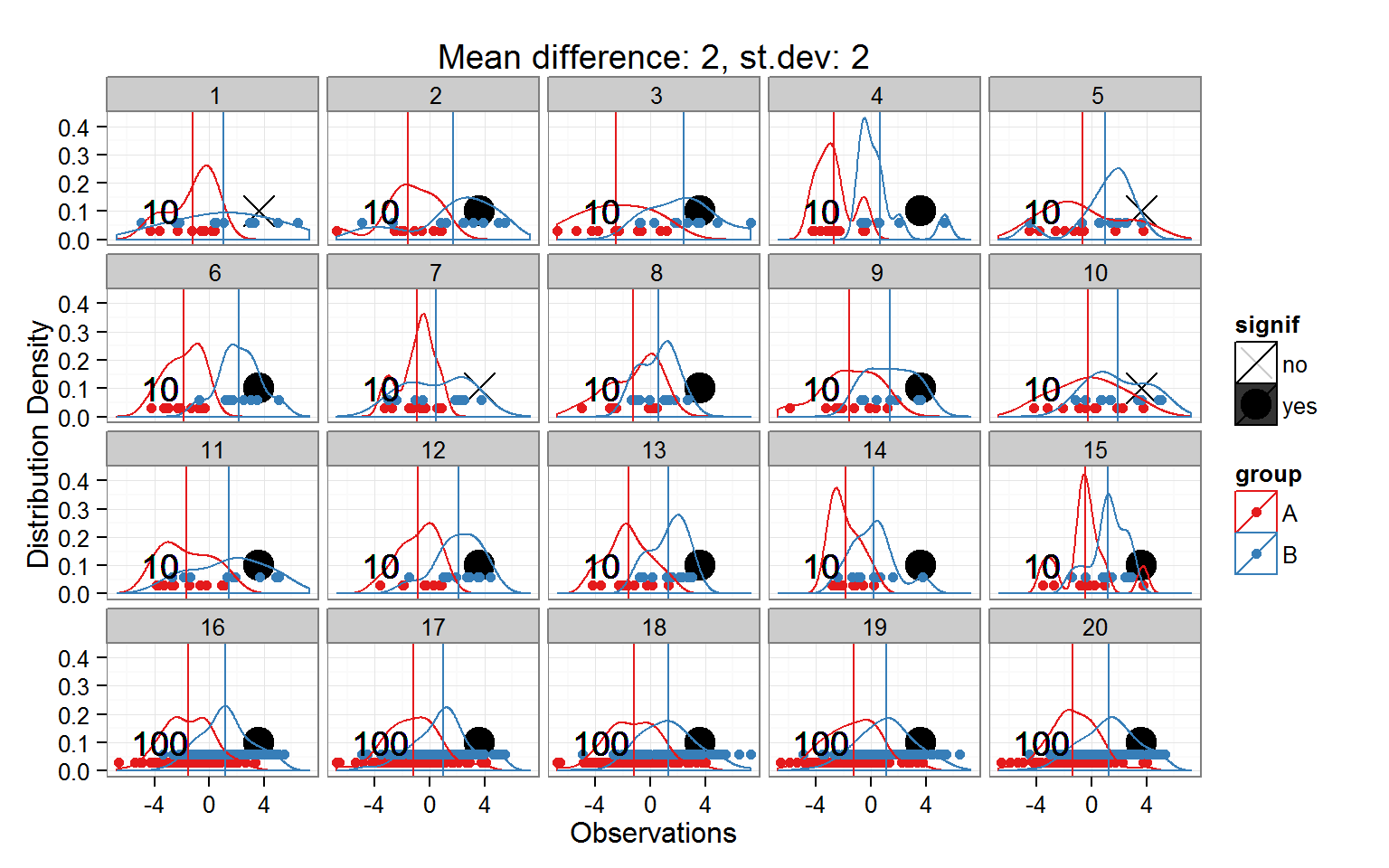Now we can see that for every one of the large-n (100 sample) distributions, the true difference was identified as significant. For some of the small-n (10 sample) distributions, sometimes the difference was missed!

This is an R Markdown document. Markdown is a simple formatting syntax for authoring HTML, PDF, and MS Word documents. For more details on using R Markdown see http://rmarkdown.rstudio.com.